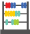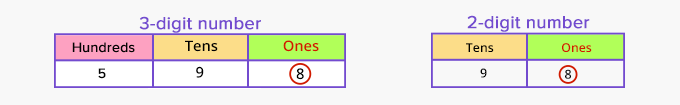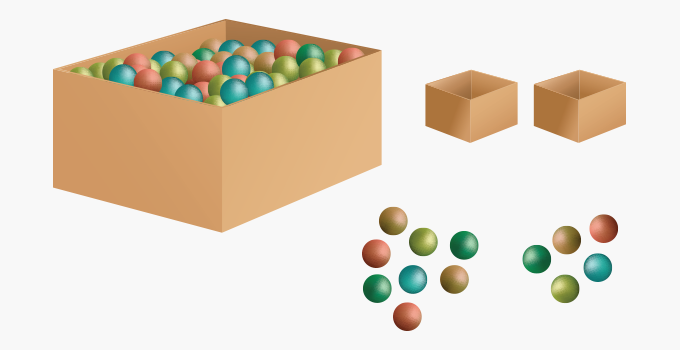# Odd Number - Definition with Examples

The Complete K-5 Math Learning Program Built for Your Child

• 30 Million Kids

Loved by kids and parent worldwide

• 50,000 Schools

Trusted by teachers across schools

• Comprehensive Curriculum

Aligned to Common Core

##Let's learn!

What are odd numbers?
Odd numbers are whole numbers that cannot be divided exactly into pairs.

Odd numbers, when divided by 2, leave a remainder of 1.1, 3, 5, 7, 9, 11, 13, 15 … are sequential odd numbers.

Odd numbers have the digits 1, 3, 5, 7 or 9 in their ones place.Fun Facts The sum of two odd numbers is always even. The product of two or more odd numbers is always odd. The sum of an even number of odd numbers is even, while the sum of an odd number of odd numbers is odd. For instance, the sum of the four odd numbers 9, 13, 21 and 17 is 60, while the sum of five odd numbers 7, 15, 19, 23 and 29 is 93.

##Let's sing!

One, three, five, seven and nine,
All standing in a straight line.
Divide them into equal teams,
One is left, and alone it seems!

##Let's do it!

Put an odd number of beads in a box. Ask your child to count and determine if the total number of beads is even or odd. Next, give your child two empty boxes. Instruct him/her to divide the beads into the two boxes such that each of them have equal number of beads. Ask how many are left.

Further, ask your child to pick out an even number of beads and then, an odd number of beads. Show that the sum or difference of an even and odd number is odd.##Related math vocabulary

Won Numerous Awards & Honors